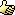1. ## Question about Lambda Expressions

I saw Lambda Expressions in a reply in the "What's New" thread, and have a few questions about it.

As I understand in Lambda Expressions were added to provide functionality for LINQ. My questions are as follows:

1) What the heck is it?
2) What would I use it for? Bonus points for explaining why I would and/or not use it in some circumstances. Or better, a situation in which I could use it, but shouldn't.
3) Could you provide an example of one? This is where I've had problems, most of the examples I've seen have been so esoteric, that I just don't get it.

-tg2. ## Re: Question about Lambda Expressions

Hi technome,

I actually had trouble learning them as well and am very thankful that Visual Basic provides the common ones you use in LINQ queries as query expressions instead. So I think I'll go for the "bonus points"...

Here's an example of a simple lambda expression, or as I like to call them, inline functions, that adds x to itself:

Code:
`Dim myFunc = Function(x) x + x`
To call this, we could write:

Code:
`Console.Writeline(myFunc(2))`
Which results: 4

Extension Methods are another new feature and allow you to create methods that extend any type. For instance you could create your own Print method on the string class. The LINQ framework relies heavily on Lambda expressions as well as Extension Methods. In fact, the LINQ syntax we see in Visual Basic is really just abstracting away Extension Methods and Lambda Expressions for us in most cases. For instance, the Sum extension method accepts a lambda expression as one of it's parameters. Say we want to only sum even numbers in an array. We can do this in one line of code using a lambda expression:

Code:
```Dim x As Integer() = {1, 2, 3, 4, 5, 6}
Dim sumofEvens = x.Sum(Function(y) If(y Mod 2 = 0, y, 0))```
Lambda functions start to get really useful (and necessary) when we start writing LINQ queries. Say we want to Sum all of the products in our products collection by multiplying the UnitPrice by the UnitsInStock. We could write this:

Code:
``` Dim total = (From Product In db.Products) _
.Sum(Function(p As Product) p.UnitPrice * p.UnitsInStock)```
But why? (I think this is my bonus point) In Visual Basic we don't have to write lambda expressions to do aggregate operations like this. (see here: http://msdn2.microsoft.com/en-us/lib...38(VS.90).aspx) We can instead write this using Visual Basic's Query Expressions:

Code:
``` Dim total = Aggregate Product In db.Products _
Into Sum(Product.UnitPrice * Product.UnitsInStock)```
I love it! Much easier to figure out what's going on IMHO. I hope that helps clear it up.

Have Fun!
-B3. ## Re: Question about Lambda Expressions

Cool deal, I like these "inline functions". Thanks for the code examples.####Posting Permissions

• You may not post new threads
• You may not post replies
• You may not post attachments
• You may not edit your posts
•